# Arrays Guide

## Array

An array is a group of items of the same type that share a common name. The elements in an array are stored at contiguous memory locations.
Each location of an element in an array is indicated by an integer known as index, which is used to identify the element.
Value of index starts from `0` and goes on till `size-1` where `size` is the number of elements present in the array.
The values in the array are indicated by writing the index or subscript within square brackets after the array name.

For example, consider an array `{2, 4, 6, 8, 10, 12}` :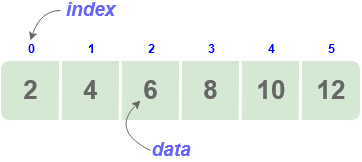Here, the array length is 6 that means it can store 6 elements.
Each element can be accessed via its index. For example, element at index 2 is `6`.

## Representation & Accessing elements in an array

Representation :

Arrays are generally represented as :

• `datatype array_name[array_size]`
• `datatype array_name[] = {value1, vlaue2, value3};`
• `datatype array_name[size] = {value1, vlaue2, value3}`

where data-type has to be a valid data type ( int, float, char…)

name must be a valid identifier

We can create arrays of various data types :

• Integer array : `int` arr[] = {10, 20, 30, 40, 50};
• Character array :`char` arr[] = {‘a’, ‘b’, ‘c’, ‘d’, ‘e’};

Accessing elements: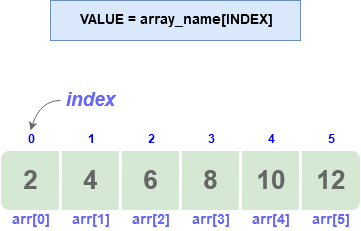To access elements in an array, the index or subscript is put within square brackets after the array name. : `array_name[index]`
For example, consider an array `arr[] = {2, 4, 6, 8, 10, 12}`
`arr` gives us the first element i.e. 2,
`arr` gives us the second element i.e. 4,
`arr` gives us the last element i.e. 12.

## Array Traversal

This operation is to traverse through the elements of an array.

``````   void Display()
{
int size  =5;
int arr[] = {2,4,6,8,10};
for(i = 0; i<size; i++)
{
print(arr[i]);
}
}
``````

## Array Insertion

### Insertion at the beginning

For inserting at the beginning of an array, the existing element need to be shifted forward.

• Assume an array arr with N elements.
• The maximum numbers of elements it can store is defined by size.
• Check if the array has space
• If yes, then proceed with the insertion operation.
• Shift the element by one index each
• insert the element at the first index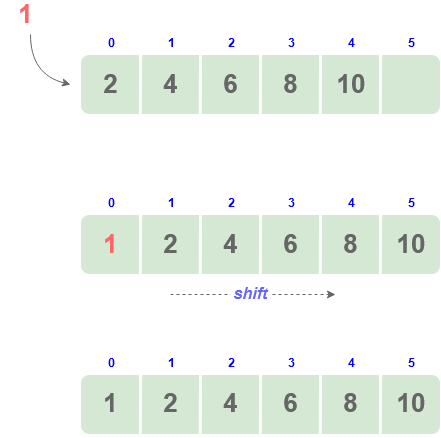Code

``````void Insert(int val)
{
//shift the elements
for(i = N; i >= 0; i--)
{
arr[i+1] = arr[i];
}
// insert the new element
arr = val;
N++;//increase current size of the array
}
``````

### Insertion at a given index

The exact location of the array where the new element needs to be inserted is provided.

• Check if the array is full
• If not, move(shift) all elements from that position one step forward to make space for the new element.
• Insert the element at the index.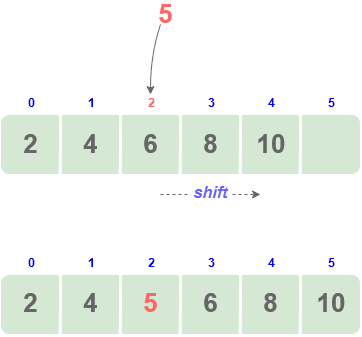Code

``````void Insert(int position,int val)
{
// Shifting rest of the elements forward
for(int i = N; i >= position; i--)
{
arr[i+1] = arr[i];
}
// add new element at given position
arr[positon] = val;
N++;
}
``````

### Insertion at the end

• Check if there is space in the array
• If yes, insert array at index-1
• Increase value of N(current size)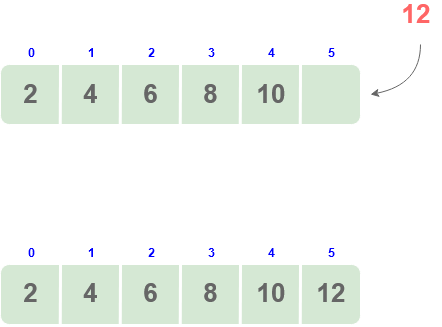Code

``````void Insert(int arr[], int size,int val,int capacity)
{

// Cannot insert more elements if n is
// already more than or equal to capcity
if (n >= capacity)
return n;

arr[n] = key;

return (n + 1);
}
``````

## Array Deletion

### Steps:

• Check if array is empty
• traverse to the position at which the element has to be deleted
• Starting from given index, shift all elements one index backwards
• decrement the value of size

For deletion at start, position = 0

#### Deletion at the beginning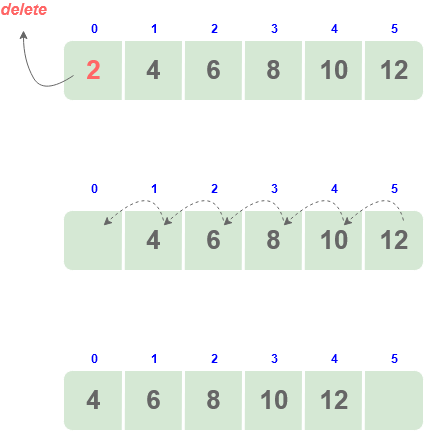### Deletion from a given index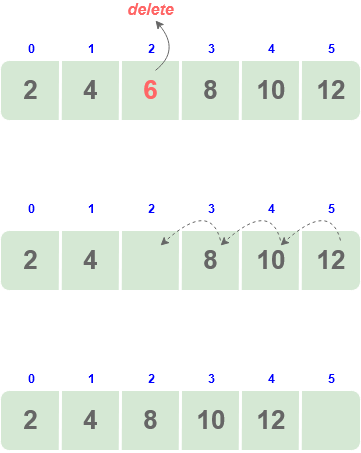### Deletion at the end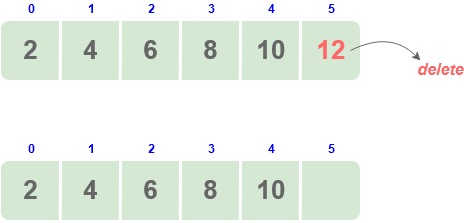Code

``````int Delete(int arr[], int pos, int n)
{

if (pos == - 1)
{
return n;
}

// Deleting element
for (int i = pos; i < n - 1; i++)
arr[i] = arr[i + 1];

return n - 1;
}
``````

## Time Complexity

### Searching

For searching any element, the entire array has to be traversed : `O(n)`

### Insertion

Inserting at the beginning of the array `O(n)`

Inserting at the end of the array `O(1)`

Inserting into the middle of an array since all the elements after that element have to be shifter , so the complexity is `O(n)`

### Deletion

Deleting an element from an array requires shifting all the elements to the left by 1. `O(n)`

Scroll to Top

### Full Stack Integrated Bootcamp Free Trial

• Please enter a number from 7000000000 to 9999999999.